This content got low rating by people.

Tokens power day 9 09/09/2021

The purpose of this account will be to store Hives as well as tokens (tokens in power)

Objectif

Alive + 3.158
total = 140.817
Objectif = 1 000
vote value = 0.079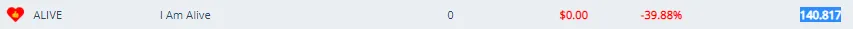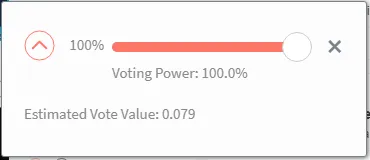Battle + 5.18
total = 1465.03
Objectif = 2 000
vote value = 0.55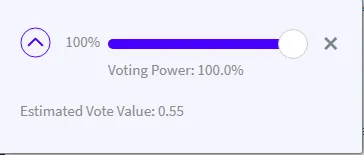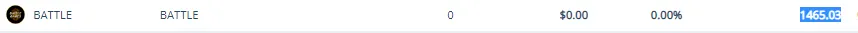Build + 10.8293504
total = 10.8293504
Objectif = 1 000
vote value = 0.00019440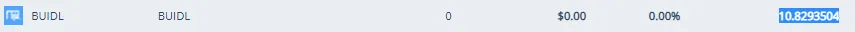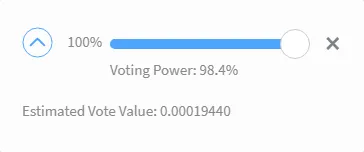POB + 0.08370019
total = 0.90780961
Objectif = 100
vote value = 0.00060392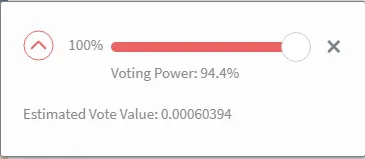cine + 0.00028454
total = 2.26106054
Objectif = 100
vote value = 0.00243353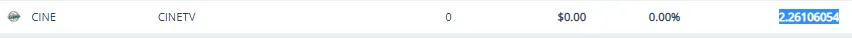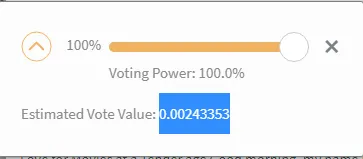Leo + 0.079
total = 1.313
Objectif = 100
vote value = 0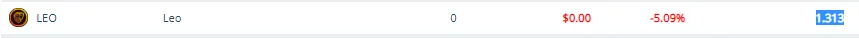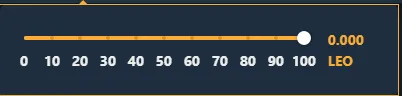Lern + 11.79898176
total = 100.72656958
Objectif = 1000
vote value = 0.52302631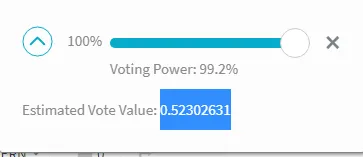BHT + 14.99582
total = 31.06377
Objectif = 1000
vote value = 0.05591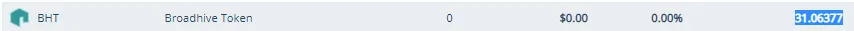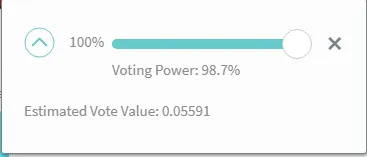Palnet + 0.005
total = 0.006
Objectif = 10
vote value = 0

H2
H3
H4
3 columns
2 columns
1 column
1 Comment